## The length of a rectangle field is represented by the expression 14 X minus 3X squared +2 Y. The width of the field is represented by the ex

Question

The length of a rectangle field is represented by the expression 14 X minus 3X squared +2 Y. The width of the field is represented by the expression 5X minus 7X squared plus 7Y. How much greater is the length of the field than the width?

in progress 0
7 months 2021-07-23T05:38:28+00:00 1 Answers 4 views 0

## Answers ( )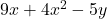Step-by-step explanation:

Hi there!

Length of the field: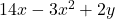units

Width of the field: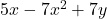units

To find how much greater the length of the field is than the width, subtract the width from the length: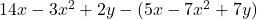Open up the parentheses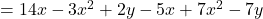Combine like terms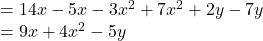Therefore, the length isunits greater than the width.

I hope this helps!